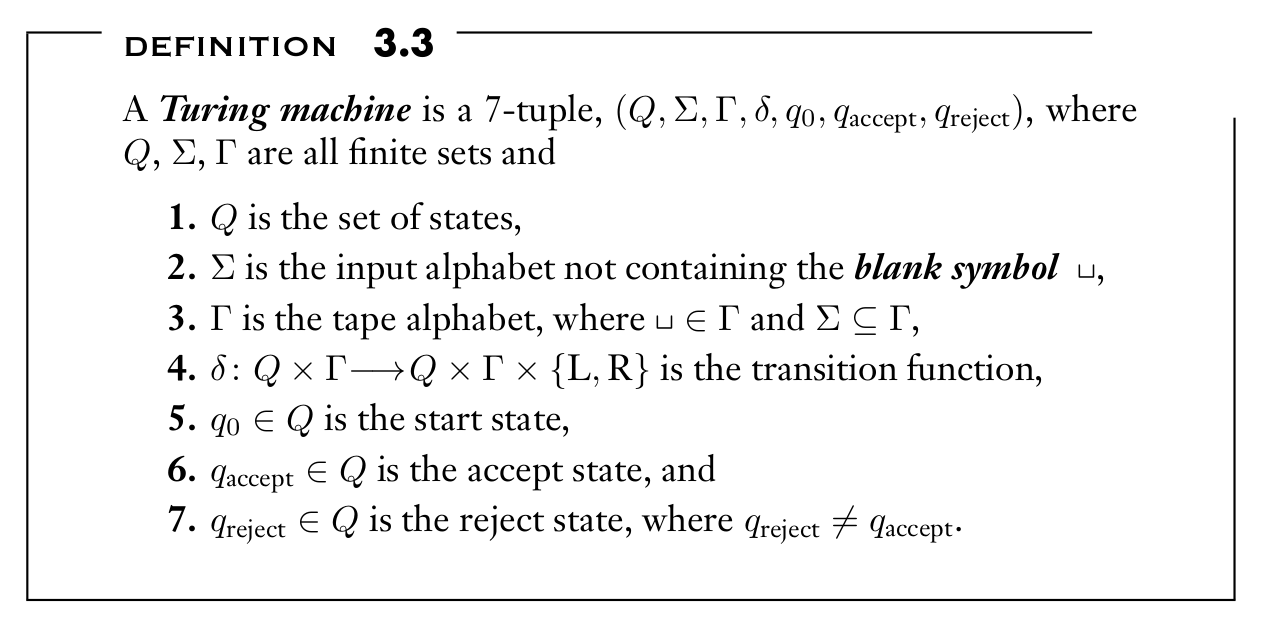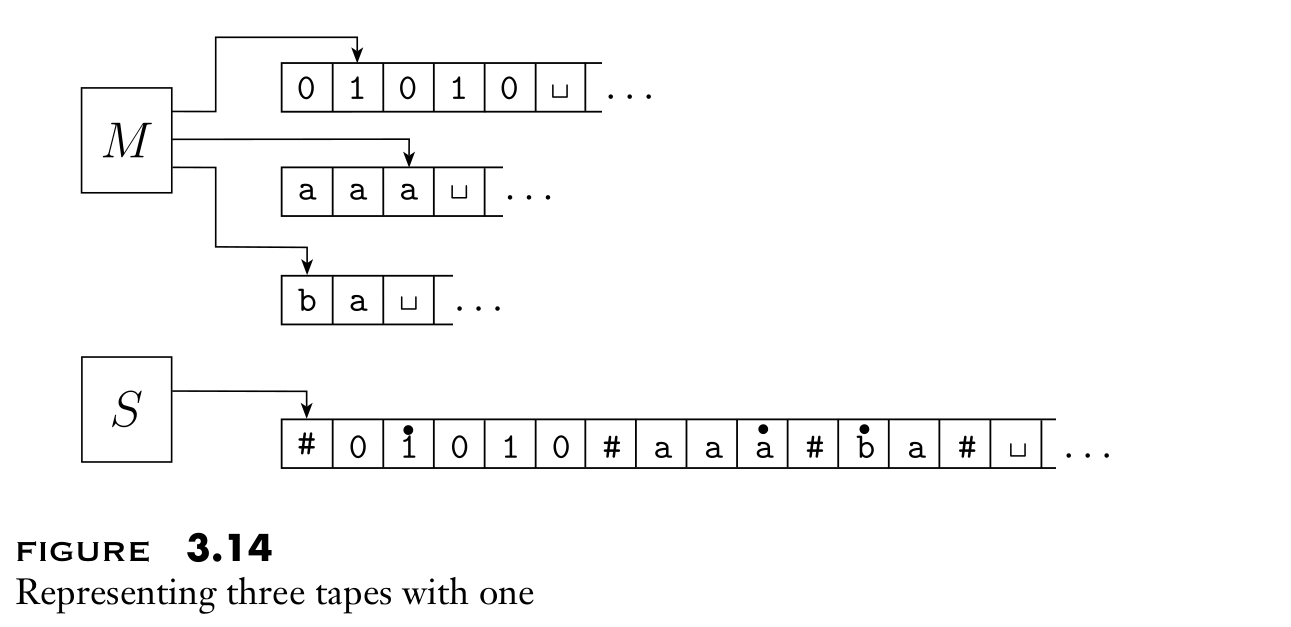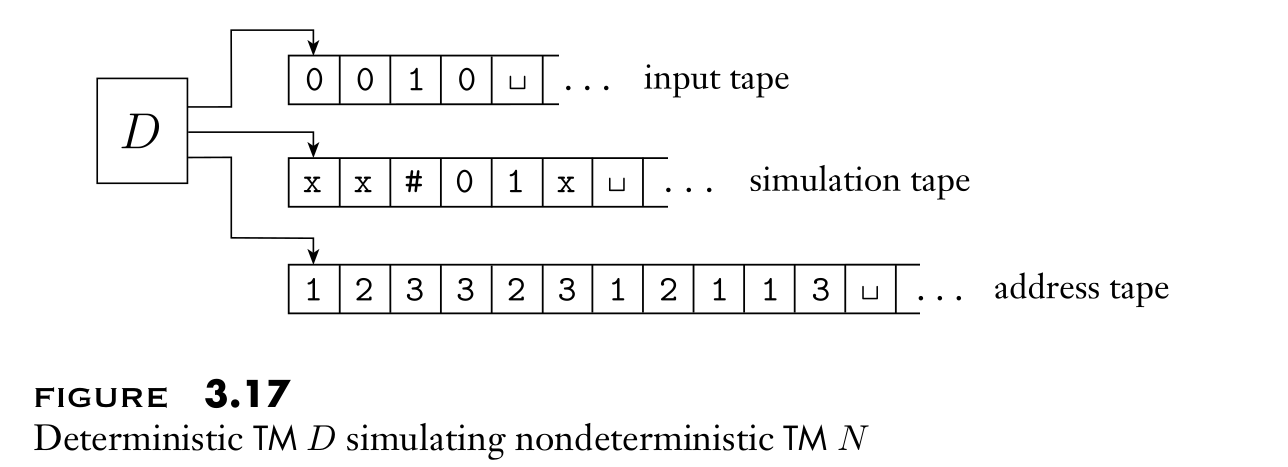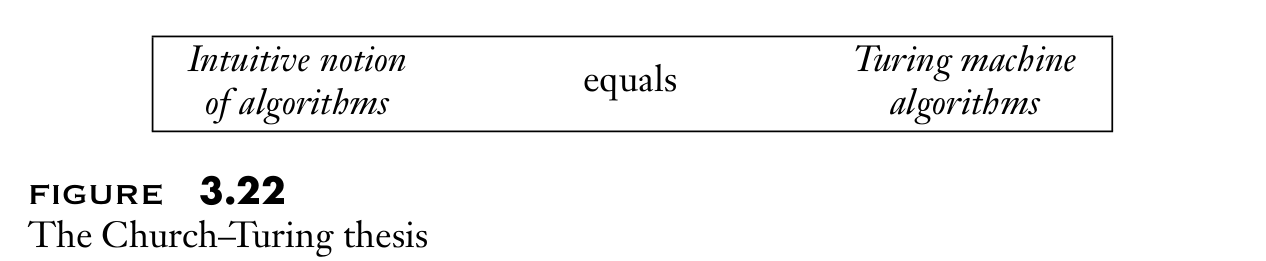[ITOC-ch3] THE CHURCH—TURING THESIS

3.1 TURING MACHINES

The Turing machine model uses an infinite tape as its unlimited memory.

It has a tape head that can read and write symbols and move both left and right on the tape.

The outputs accept and reject are obtained by entering designated accepting and rejecting states. If it doesn’t enter an accepting or a rejecting state, it will go on forever, never halting.

DEFconfiguration

• a setting of current state, the current tape contents, and the current head location.

Machine M

Recognizer

• input a w, accept, reject or loop
• may fail to accept an input by entering the $q_{reject}$ state and rejecting, or by looping

EX:

if $w \in L$, than M must accept w.

if $w \notin L$, than M may loop when input w.

Decider

• halts on all inputs

EX:

if $w \in L$, than M must accept w.

if $w \notin L$, than M must reject w.

Language L

The collection of strings that M accepts

Turing-recognizable language

• there exist some Turing machine recognizes it.

Turing-decidable language

• there exist some decider decides it.

?Undecidable language

• exist recognizer accept all strings $w \in L$

• No decider exist, always loop when input specific string $w \notin L$

example: 4.2

?H can’t halt when its input D simulate H itself

3.2 VARIANTS OF TURING MACHINES

MULTITAPE TURING MACHINES

$δ : Q × Γ^k → Q × Γ^k × { \{L, R, S\} }^k$

equivalent with ordinary Turing machine

• uses # to separate different tapes
• uses dot to keep track the headsNONDETERMINISTIC TURING MACHINES

$δ : Q × Γ→P(Q × Γ × \{L, R\})$

equivalent with ordinary Turing machine

• have D try all possible branches of N ’s nondeterministic computation
• tape 1: input; tape2: copy of N ’s tape on some branch;
• tape 3: node address of N where D is now inENUMERATORS

• a Turing machine with an attached printer
• The language enumerated by E is the collection of all the strings that it eventually prints out.

equivalent with ordinary Turing machine

• construct M recognize language A enumerated by E
• construct E enumerate language A recognized by M

3.3 THE DEFINITION OF ALGORITHM

Informally speaking, an algorithm is a collection of instructions to perform some task.

HILBERT’S PROBLEMS

Algorithm

DEF

1. λ-calculus notation system by Alonzo Church
2. Turing machines by Alan Turing

Church–Turing thesisExample: Hilbert’s tenth problem

• Algorithm: Test whether a polynomial has an integral root
• TM: constructing such algorithm equals constructing a TM to decide the language D

$D = \{p \ | \ p \ is \ a \ polynomial \ with \ an \ integral \ root\}.$

• decider D can’t exist, problem algorithmically unsolvable.

TERMINOLOGY FOR DESCRIBING TURING MACHINES

3 levels describing Algorithm or TM

• formal description
• spells out in full the Turing machine’s states, transition function, and so on
• implementation description
• describe the way TM moves its head and the way it stores data on its tape
• high-level description
• input encoded object as strings
• break the algorithm into stages, each involves individual steps of the Turing machine’s computation